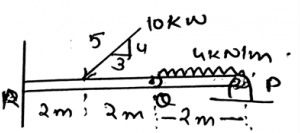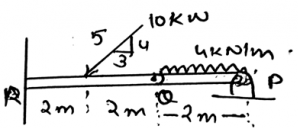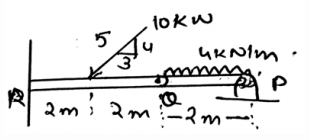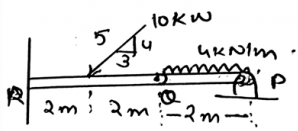Courses

# Test: Frame And Machines

## 15 Questions MCQ Test Engineering Mechanics | Test: Frame And Machines

Description
This mock test of Test: Frame And Machines for Mechanical Engineering helps you for every Mechanical Engineering entrance exam. This contains 15 Multiple Choice Questions for Mechanical Engineering Test: Frame And Machines (mcq) to study with solutions a complete question bank. The solved questions answers in this Test: Frame And Machines quiz give you a good mix of easy questions and tough questions. Mechanical Engineering students definitely take this Test: Frame And Machines exercise for a better result in the exam. You can find other Test: Frame And Machines extra questions, long questions & short questions for Mechanical Engineering on EduRev as well by searching above.
QUESTION: 1

### Frames and machines are two types of structural figures which are often composed of pin connected ___________ member.

Solution:

Frames and machines are two types of structural figures which are often made of pin connected multi-force members. That is more than two forces are subjected over the machines. Thus the term multi-force is used for the frames and the machines.

QUESTION: 2

### ___________ are used as support loads.

Solution:

The frames are used as support for the structures. They hold up the structure into the right place. They are made for holding up the structures in the place. They also provide the stability to the structure.

QUESTION: 3

### Find the horizontal reaction at Q.Solution:

The machines are designed such that they can transmit the effects of forces. Thus making the free body diagrams of the various joints and doing the calculations makes us determine the various reaction values. And for the asked reaction equating the forces acting equal to zero, we get the answer.

QUESTION: 4

________ contains moving parts.

Solution:

The machines are designed such that they contain the moving parts. As the machines are made for working in various fields, they are designed as to contain the moving parts. They are also such designed such that the moving parts in are not damaged.

QUESTION: 5

Machines are so designed to ___________ and alter the effects of the forces.

Solution:

The machines are designed such that they can transmit the effects of forces. As the machines are made for working in various fields, they are also such designed so as to alter the effects of the forces. They are also such designed such that the moving parts in are not damaged.

QUESTION: 6

Find the horizontal reaction at R.Solution:

The machines are designed such that they can transmit the effects of forces. Thus making the free body diagrams of the various joints and doing the calculations makes us determine the various reaction values. And for the asked reaction equating the forces acting equal to zero, we get the answer.

QUESTION: 7

The forces acting on the joints and on the members are determined by the simple equilibrium equations, which are used in the usual beams and trusses.

Solution:

The forces acting on the joints and on the members are determined by the simple equilibrium equations, which are used in the usual beams and trusses. The forces which are acting over the joint of the frames and machines are no more different from the forces acting on the beams and trusses.

QUESTION: 8

Forces acting on the supports and the joints are not important to determine, in the frames and machines.

Solution:

The forces are determined because the designing of the frames and machines. This is done so as if the forces are applied to frames and the machines, they don’t collapse. Thus the forces acting on the joints and the members are important to be determined.

QUESTION: 9

The forces obtained at the joints and the members of the machines are very important. They are used for:

Solution:

The forces obtained at the joints and the members of the machines are very important. They are used for the designing the size of the members, connections done in the frames and machines. They are obtained by applying the simple equilibrium force equations on the system. Net forces are made to be zero, and the unknowns are determined.

QUESTION: 10

There are no free body diagram used in the calculations of the forces at the supports and at the joints. Because the free body diagrams of a machines are so hard to be made.

Solution:

There is a great need of the making of the free body diagrams in the calculations of the forces that are being acted upon the joints. This helps in making of the design of the machines and the frames. These are very important quantities that are being determined.

QUESTION: 11

Which of the following is correct for the frames in 2D and machines in 3D?

Solution:

The application of the conditions of the equilibrium of the body is valid throughout. This means that the conditions are irrespective of the dimensions. The conditions are the basic rules that defines the equilibrium of the body and thus are applicable in any dimension of the real axis.

QUESTION: 12

Find the vertical reaction at R.Solution:

The machines are designed such that they can transmit the effects of forces. Thus making the free body diagrams of the various joints and doing the calculations makes us determine the various reaction values. And for the asked reaction equating the forces acting equal to zero, we get the answer.

QUESTION: 13

If a force applied at any point in its line of action and is still creating the same moment about any fixed point say P, then the force is said to be______________

Solution:

If a force applied at any point in its line of action and is still creating the same moment about any fixed point say P, then the force is said to be sliding vector. This is because the moment of the force which is acting on its line of axis at the point P is same throughout. Whatever be the direction of the distance.

QUESTION: 14

If a force applied at any point in its line of action and is still creating the same moment about any fixed point say P, then the force is said to be sliding vector. What is the name of this property?

Solution:

If a force applied at any point in its line of action and is still creating the same moment about any fixed point say P, then the force is said to be sliding vector. This is because the moment of the force which is acting on its line of axis at the point P is same throughout. This is known as the principle transmissibility of the force.

QUESTION: 15

Find the vertical reaction at Q.Solution:

The machines are designed such that they can transmit the effects of forces. Thus making the free body diagrams of the various joints and doing the calculations makes us determine the various reaction values. And for the asked reaction equating the forces acting equal to zero, we get the answer.